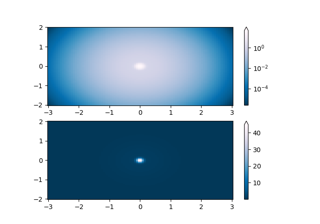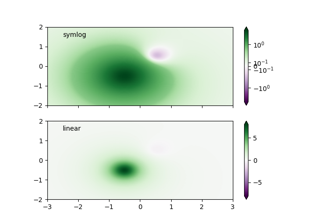# matplotlib.colors.SymLogNorm#

class matplotlib.colors.SymLogNorm(linthresh, linscale=1.0, vmin=None, vmax=None, clip=False, *, base=10)[source]#

Bases: `SymLogNorm`

The symmetrical logarithmic scale is logarithmic in both the positive and negative directions from the origin.

Since the values close to zero tend toward infinity, there is a need to have a range around zero that is linear. The parameter linthresh allows the user to specify the size of this range (-linthresh, linthresh).

Parameters:
linthreshfloat

The range within which the plot is linear (to avoid having the plot go to infinity around zero).

linscalefloat, default: 1

This allows the linear range (-linthresh to linthresh) to be stretched relative to the logarithmic range. Its value is the number of decades to use for each half of the linear range. For example, when linscale == 1.0 (the default), the space used for the positive and negative halves of the linear range will be equal to one decade in the logarithmic range.

basefloat, default: 10
Parameters:
vmin, vmaxfloat or None

If vmin and/or vmax is not given, they are initialized from the minimum and maximum value, respectively, of the first input processed; i.e., `__call__(A)` calls `autoscale_None(A)`.

clipbool, default: False

If `True` values falling outside the range `[vmin, vmax]`, are mapped to 0 or 1, whichever is closer, and masked values are set to 1. If `False` masked values remain masked.

Clipping silently defeats the purpose of setting the over, under, and masked colors in a colormap, so it is likely to lead to surprises; therefore the default is `clip=False`.

Notes

Returns 0 if `vmin == vmax`.

__call__(value, clip=None)[source]#

Normalize value data in the `[vmin, vmax]` interval into the `[0.0, 1.0]` interval and return it.

Parameters:
value

Data to normalize.

clipbool

If `None`, defaults to `self.clip` (which defaults to `False`).

Notes

If not already initialized, `self.vmin` and `self.vmax` are initialized using `self.autoscale_None(value)`.

autoscale_None(A)[source]#

If vmin or vmax are not set, use the min/max of A to set them.

inverse(value)[source]#

## Examples using `matplotlib.colors.SymLogNorm`#Colormap Normalizations

Colormap NormalizationsColormap Normalizations SymLogNorm

Colormap Normalizations SymLogNormColormap Normalization

Colormap Normalization